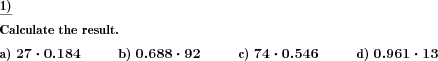Custom math worksheets at your fingertips# Details for problem "Decimal fraction multiply by whole number"

Quickname: 9400

Elementary School, Primary School, Junior High School, Middle School, High School.

## Summary

A decimal fraction has to be multiplied by a whole number.

## Example## Description

A decimal fraction is one of the basic topics to learn in Maths and this worksheet provides practice problems related to decimal fractions divided by a whole number. A decimal fraction is presented, it is one factor in a multiplication problem. The other factor is a whole number. Practicing these worksheets is necessary for learners to grasp the multiplication of decimal fractions with whole number skills. Assign these problems to struggle students for independent practice/ homework and let them grasp the skills. These worksheets can also be used in variation for assessment purposes. Along with this main objective, grade 6 to grade 8 learners will also achieve the following set of targets.

1. Revise the concepts of multiplication.
2. Reinforce the concept of multiplication with a whole number.
3. Use mental maths times table skills.
4. Master the multiplication of a decimal fraction with a whole number.
5. Apply this skill to real-world problems.

Personalize these worksheets and serve to differentiated ability learners.

• The number of places before the decimal point can be selected.
• The number of decimals can be selected for the decimal fraction factor.
• In addition, the first n decimal places can be made with zero digits. In this way, several different shapes can be generated.
• For the other factor, the maximum power of ten applied can be defined.

The number of problems can be selected.

Some examples:

Number of places: 0 Number of decimals: 3 Leading decimals=0: 0

```    0.222 * 22      0.456 * 12      0.999 * 10
```

Number of places: 2 Number of decimals: 2 Leading decimals=0: 0

```    12.21 * 22      89.12 * 12      23.44 * 10
```

Number of places: 0 Number of decimals: 3 Leading decimals=0: 2

```    0.002 * 22      0.001 * 12      0.008 * 10
```

The answer key consists of all problems with solutions.

Download free printable worksheets for this math problem here. The worksheet contains the problems only, the solution sheet includes the answers. Just click on the respective link.

•Worksheet 1Solution sheet with answers
•Worksheet 2Solution sheet with answers
•Worksheet 3Solution sheet with answers

If you can not see the solution sheets for download, they may be filtered out by an ad blocker that you may have installed. If this is the case, please allow ads for this page and reload the page. The solution sheets will then reappear.

• Do these sample worksheets do not really fit?
• Do you need more math worksheets, with a different level of difficulty?
• Would you like to combine different problems on a worksheet and adjust them to your needs?
• As a teacher, you can put together your own worksheets using the automatically generated math problems provided.
With a free initial credit, you can start creating your own math worksheets in a few minutes.

You can try it for free! Register here, to create custom worksheets now!

## Customization options for this problem

Parameter
Possible values
Number of problems
1, 2, 3, 4, 5, 6, 7, 8, 9, 10
Places
0, 1, 2, 3
Decimal places
1, 2, 3, 4
Decimals 0 up to pos
0, 1, 2, 3
Number range whole no
10, 20, 30, 40, 50, 100, 200, 500, 1000, 10000, 100000
Swap factors
Yes, No